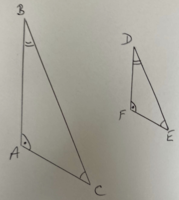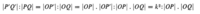# Similar triangles and inversion

#### Mondo

##### New member
I have some doubts regarding to few fragments of a book:
1. "The segment inverts into a circular arc (Fig 23.1) - do you guys see an arc there? I don't
2. If O is the center of inversion the triangles OPQ, OQ'P' are similar and for example author writes:
$$\displaystyle OP \cdot OP' = OP \cdot OQ$$ -> I see they multiply segments with common angles but the inspection of a triangles clearly shows that OP' is much much longer than OQ so this equality can not be true right?
Also, did he assumed the angles shown on the Figure below or this is a property of inversion?Thanks

#### skeeter

##### Elite Member
$$\displaystyle \Delta OQP \sim \Delta OP’Q’$$ by the Angle-Angle theorem

the corresponding sides of similar triangles are proportional, therefore the product should be

$$\displaystyle \frac{OP}{OQ’} = \frac{OQ}{OP’} \implies OP \cdot OP’ = OQ \cdot OQ’$$

maybe a typo omission of the prime?

can’t answer your query about the “inversion” … I’d have to study up on that concept.

•Mondo

#### lex

##### Full Member
•Mondo

#### Mondo

##### New member
@lex yes, I by mistake put equal sign instead of a colon. Anyway, these proportions are still a bit awkward to me. I am used to ratios of two similar triangles A and B like "one side of A": "other side of A" = "one side of B" : "other side of B" but here they compare sides from two different triangles. I am especially confused about the ratio I mentioned in the opening post, namely: OP.OP':OP.OQ -> here they multiply one side of a small triangle with a fragment of the larger triangle side and relate it to the product of two sides of a small triangle. How can we prove that this ration is the same as the others given earlier in the line (which seem more logical)?

#### lex

##### Full Member$$\displaystyle \bigtriangleup$$ ABC is similar to $$\displaystyle \bigtriangleup DEF$$

So $$\displaystyle \frac{AB}{AC} \equiv \frac{FD}{FE}$$ is what you are used to

but this $$\displaystyle \leftrightarrow \frac{FE}{AC} \equiv \frac{FD}{AB}$$ which you are not used to!
In many ways, I find the second way more understandable, as the lengths in one shape are the same fraction of the lengths in the other shape, and this ratio represents just that fraction. E.g. the lengths in the second triangle might be 1/2 of the corresponding lengths in the first triangle. Therefore if you take the ratio of corresponding sides in the two shapes you should always get the same ratio, namely 1:2 or $$\displaystyle \frac{1}{2}$$

Next, this line:The first part is simply comparing corresponding sides in the two similar triangles:
$$\displaystyle |P'Q'| : |PQ| \equiv |OP'|:|OQ| \hspace1ex$$ (1)

They now simply multiply both parts of the ratio on the right hand-side by |OP| and therefore get an equivalent ratio -

$$\displaystyle (|P'Q'| : |PQ|) \hspace2ex \equiv \hspace2ex |OP'|:|OQ| \hspace2ex \equiv \hspace2ex |OP|.|OP'|:|OP|.|OQ| \hspace1ex$$ (2)
(They could have multiplied by any positive number, but they chose |OP|)

Finally they use the fact that |OP|.|OP'| is $$\displaystyle k^2$$ (since P' is the inverse of P in the circle) to rewrite the left-hand part of the final ratio in (2):

$$\displaystyle (|P'Q'| : |PQ| \hspace2ex \equiv \hspace2ex |OP'|:|OQ| \hspace2ex \equiv \hspace2ex |OP|.|OP'|:|OP|.|OQ|) \hspace2ex \equiv \hspace2ex |OP|.|OP'|:|OP|.|OQ|\hspace2ex \equiv k^2 : |OP|.|OQ| \hspace1ex$$ (3)

•Mondo

#### Mondo

##### New member
Ok I see. I think I was too focused on geometrical relations so I miss the clear algebraic multiplication...thanks Lex!

PS: Your right angles look way to obtuse#### lex

##### Full Member
Ok I see. I think I was too focused on geometrical relations so I miss the clear algebraic multiplication...thanks Lex!

PS: Your right angles look way to obtuseYes. That makes sense.
(They aren't supposed to be right angles! My little invented symbol was to show two angles that are the same!)

•Mondo